﻿ NLVM 6 - 8 - Number & Operations Manipulatives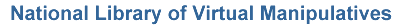Number & Operations (Grades 6 - 8)

Virtual manipulatives for Number & Operations, grades 6 - 8.Abacus – An electronic abacus that can be used to do arithmetic.Base Blocks – Illustrate addition and subtraction in a variety of bases.Base Blocks Addition – Use base ten blocks to model grouping in addition.Base Blocks Decimals – Add and subtract decimal values using base blocks.Base Blocks Subtraction – Use base ten blocks to model separation of groups in subtraction.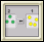Chip Abacus – Learn about carrying and digits using chips.Circle 0 – A puzzle involving adding positive and negative integers to sum to zero.Circle 21 – A puzzle involving adding positive and negative integers to sum to twenty one.Circle 3 – A puzzle involving adding positive real numbers to sum to three.Circle 99 – A puzzle involving adding positive and negative integers to sum to ninety nine.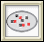Color Chips - Subtraction – Use color chips to illustrate subtraction of integers.Conway's Game of Life – Discover the rules that determine change in these simulations.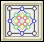Diffy – Solve an interesting puzzle involving the differences of given numbers.Dueling Calculators – Visualize a dramatic simulation of the effect of propagating rounding errors.Factor Tree – Factor numbers using a tree diagram.Fibonacci Sequence – Explore the Fibonacci sequence and the golden ratio.Fraction Pieces – Work with parts and wholes to learn about fractions.Fractions - Adding – Illustrates what it means to find a common denominator and combine.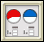Fractions - Comparing – Judge the size of fractions and plot them on a number line.Fractions - Equivalent – Illustrates relationships between equivalent fractions.Fractions - Rectangle Multiplication – Visualize and practice multiplying fractions using an area representation.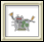Function Machine – Explore the concept of functions by putting values into this machine and observing its output.Golden Rectangle – Illustrates iterations of the Golden Section.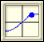Grapher – A tool for graphing and exploring functions.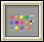Mastermind – Use inference and logic to play a game and guess a hidden pattern of pegs.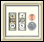Money – Learn about money by counting and making change.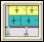Number Line Bars - Fractions – Divide fractions using number line bars.Number Line Bounce – Number line addition and subtraction game.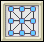Number Puzzles – Solve puzzles involving arranging numbers on a diagram so that they add up to a given value.Pascal's Triangle – Explore patterns created by selecting elements of Pascal's triangle.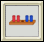Peg Puzzle – Win this game by moving the pegs on the left past the pegs on the right.Percent Grids – Represent, name, and explore percentages using hundreds grids.Percentages – Discover relationships between fractions, percents, and decimals.Sieve of Eratosthenes – Relate number patterns with visual patterns.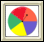Spinners – Work with spinners to learn about numbers and probabilities.Tangrams – Use all seven Chinese puzzle pieces to make shapes and solve problems.Tight Weave – Visualize the creation of the Sierpinski Carpet, an iterative geometric pattern that resembles a woven mat.Turtle Geometry – Explore numbers, shapes, and logic by programming a turtle to move.Venn Diagrams – Investigate common features of sets.